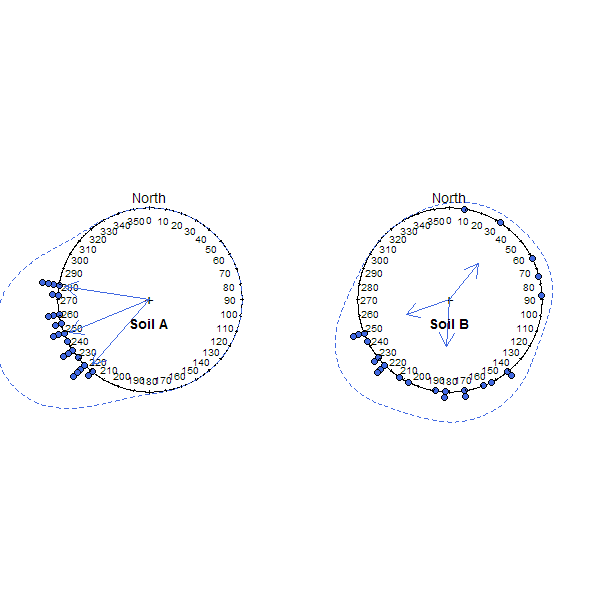aspect.plot {sharpshootR} R Documentation

## Plot Aspect Data

### Description

Plot a graphical summary of multiple aspect measurements on a circular diagram.

### Usage

```aspect.plot(p, q=c(0.05, 0.5, 0.95), p.bins = 60, p.bw = 30, stack=TRUE,
p.axis = seq(0, 350, by = 10), plot.title = NULL,
line.col='RoyalBlue', line.lwd=1, line.lty=2,
arrow.col=line.col, arrow.lwd=1, arrow.lty=1,
arrow.length=0.15,
...)
```

### Arguments

 `p` a vector of aspect angles in degrees, measured clock-wise from North `q` a vector of desired quantiles `p.bins` number of bins to use for circular histogram `p.bw` bandwidth used for circular density estimation `stack` TRUE/FALSE, should the individual points be stacked into `p.bins` number of bins and plotted `p.axis` a sequence of integers (degrees) describing the circular axis `plot.title` an informative title `line.col` density line color `line.lwd` density line width `line.lty` density line line style `arrow.col` arrow color `arrow.lwd` arrow line width `arrow.lty` arrow line style `arrow.length` arrow head length `...` further arguments passed to `plot.circular`

### Details

Spread and central tendency are depicted with a combination of circular histogram and kernel density estimate. The circular mean, and relative confidence in that mean are depicted with an arrow: longer arrow lengths correspond to greater confidence in the mean.

### Note

Manual adjustment of `p.bw` may be required in order to get an optimal circular density plot. This function requires the package `circular`, version 0.4-7 or later.

D.E. Beaudette

### Examples

``````# simulate some data
p.narrow <- runif(n=25, min=215, max=280)
p.wide <- runif(n=25, min=0, max=270)

# set figure margins to 0, 2-column plot
par(mar=c(0,0,0,0), mfcol=c(1,2))

# plot
aspect.plot(p.narrow, p.bw=10, plot.title='Soil A', pch=21, col='black', bg='RoyalBlue')
aspect.plot(p.wide, p.bw=10, plot.title='Soil B', pch=21, col='black', bg='RoyalBlue')
``````[Package sharpshootR version 0.8-4 Index]